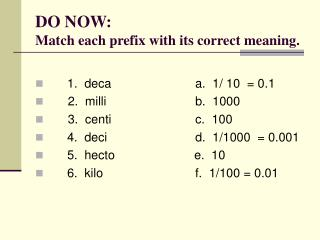Download PresentationDO NOW: Match each prefix with its correct meaning.# DO NOW: Match each prefix with its correct meaning. - PowerPoint PPT Presentation

Download Presentation##### DO NOW: Match each prefix with its correct meaning.

Download Policy: Content on the Website is provided to you AS IS for your information and personal use and may not be sold / licensed / shared on other websites without getting consent from its author. While downloading, if for some reason you are not able to download a presentation, the publisher may have deleted the file from their server.

- - - - - - - - - - - - - - - - - - - - - - - - - - - E N D - - - - - - - - - - - - - - - - - - - - - - - - - - -
##### Presentation Transcript

1. DO NOW:Match each prefix with its correct meaning. • 1. deca a. 1/ 10 = 0.1 • 2. milli b. 1000 • 3. centi c. 100 • 4. deci d. 1/1000 = 0.001 • 5. hecto e. 10 • 6. kilo f. 1/100 = 0.01

2. Temperature

3. What is the temperature? The temperature of an object describes how hot or cold the object is relative to other objects.

4. Temperature scales Scientists use two temperature scales to measure temperature. * the Celsius scale (°C) * the Kelvin scale (K)

5. Celsius Scale The Celsius scale will be used in most of your experiments. On the Celsius scale, the freezing point of water is defined as 0°C and the boiling point of water is defined as 100°C.

6. Kelvin Scale A Kelvin is the SI base unit of temperature. On the Kelvin scale, water freezes at about 273K and boils at about 373K.

7. Temperature conversions To convert from K  °C * K – 273 = °C To convert from °C  K * °C + 273 = K

8. Practice Problems Convert each temperature from °C to K. °C + 273 = K a. 54 °C = __________ K b. 69 °C= __________ K c. 15 °C= __________ K

9. Practice Problems Convert each temperature from K to °C. K – 273 = °C a. 32 K = __________ °C b. 0 K = __________ °C c. 281 K = __________ °C

10. Scientific Notation Extremely small and extremely large numbers can be compared more easily when they are converted into a form called scientific notation.

11. Expressing Quantities in Scientific Notation The surface area of the Pacific Ocean (the largest ocean) is 166,000,000,000,000 m2. (That’s 166 trillion square meters.) This quantity in scientific notation is expressed as 1.66 x 1014.

12. Steps to write scientific notation: • Move the decimal point right or left to produce a factor that is between 1 and 10. • Count the number of places you moved the decimal point. This number is the exponent (n). • Multiply the factor by 10n. • When the decimal point moves to the left, n is positive. When the decimal point moves to the right, n is negative.

13. Practice Problems a. 50,000 m/s2 =

14. Practice Problems b. 0.00000000062 kg =

15. Adding / subtracting using scientific notation To add or subtract quantities written in scientific notation, the quantities must have the same exponent. If two quantities are expressed by different powers of ten, you must change one of the quantities so that they are both expressed to the same power of ten before you add you subtract.

16. Practice Problems a. 5.10 x 1020 + 4.11 x 1021

17. Practice Problems b. 6.2 x 108 - 3. 0 x 106

18. Multiplying / dividing using scientific notation When multiplying or dividing quantities written in scientific notation, the quantities do not have to have the same exponent. For multiplication, multiply the factors, then add the exponents. For division, divide the factors, then subtract the exponents.

19. Practice Problems a. (12 x104m)(4 x 10-2m)

20. Practice Problems d. (90 x 1014kg) / (9 x 1012kg)